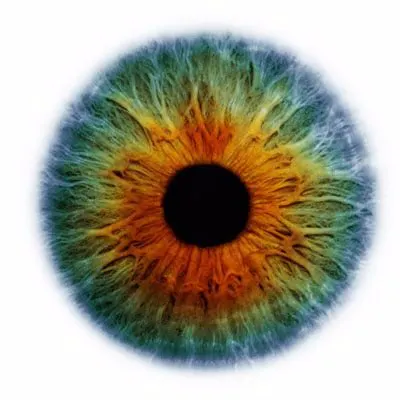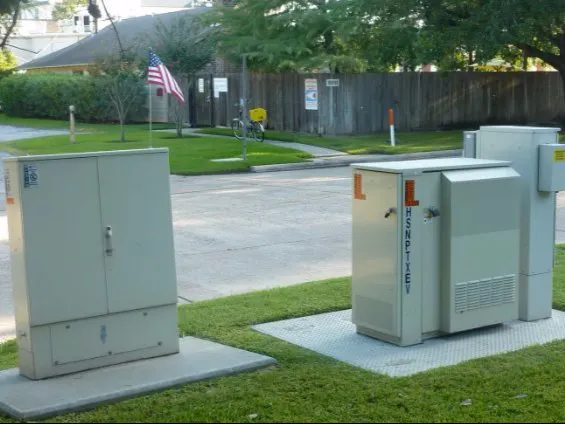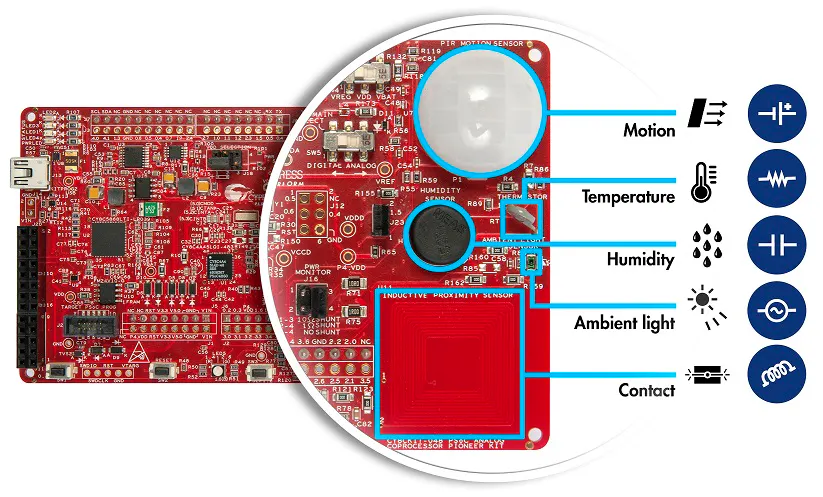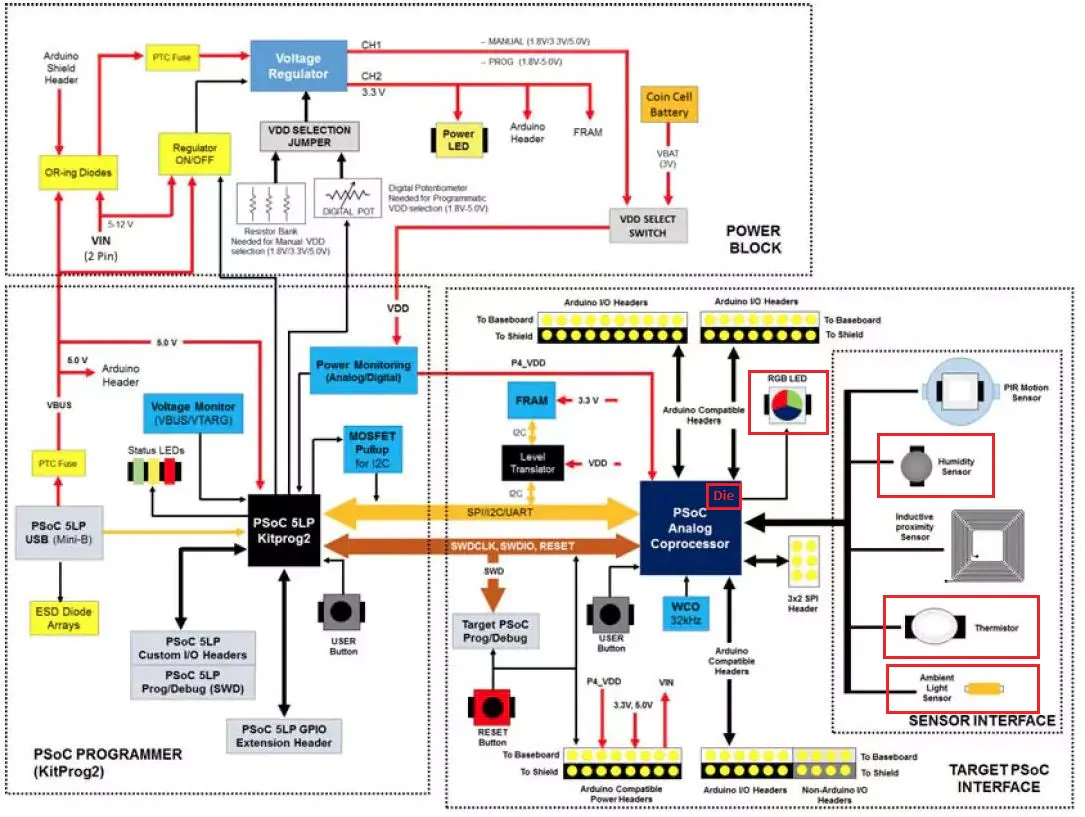Published

# Electric Box Supervisor

I would like to use the Analog Coprocessor Pioneer Kit to create the supervisor for the electric box for outdoor usage.

BeginnerFull instructions provided8 hours259## Things used in this project

### Hardware componentsCypress PSoC Analog Coprocessor Pioneer Kit
×1

## Schematics

### Electric Box Supervisor

In the red box I highlight the components which I have used.## Code

### Electric_Supervisor

C/C++
Main code for the Electric Supervisor Box project
```/******************************************************************************
* Project Name      : Electric Box Supervisor
* Version           : 1.0
* Device Used       : CY8C4A45LQI-L483
* Software Used     : PSoC Creator 4.0 update 1
* Compiler Used     : ARM GCC 4.9.3
* Related Hardware  : CY8CKIT-048 PSoC Analog Coprocessor Pioneer Kit
*******************************************************************************/

#include "project.h"
#include "math.h"

//

#define FILTER_COEFFICIENT_TEMPERATURE	(32u)

#define CAPACITANCE_AT_55_RH            (1800)

/* Sensitivity numerator and denominator indicate sensitivity of the sensor */
#define SENSITIVITY_NUMERATOR           (31)
#define SENSITIVITY_DENOMINATOR         (100)
/* Value of reference capacitor.  Note that this value includes the pin capacitance
and the physical 180pF reference capacitor */
#define CREF                            (1930)
/* Offset Capacitance */
#define COFFSET                         (150)
/* This is raw count equivalent to trace capacitance */
#define OFFSETCOUNT                     (1536)
#define BUFFERSIZE                      (8)
/* Nominal humidity 55% */
#define NOMINAL_HUMIDITY                (550)
#define HUMIDITY_0_PERCENT              (0)
#define HUMIDITY_100_PERCENT            (1000)
#define HUMIDITY_50                     (500)

#define FILTER_COEFFICIENT_ALS	        (10)

/* Constants for photodiode current calculation */
/* Scale Factor = (VREF / (2048 * 220K)) * 10^9 nA = 2.6633
As the TIA produces a negative voltage, the scale factor is made
negative */
#define ALS_CURRENT_SCALE_FACTOR_NUMERATOR		(-26633)
#define ALS_CURRENT_SCALE_FACTOR_DENOMINATOR	(10000)

/* Constants for ambient light calculation */
/* Scale Factor = 10000Lx / 3000nA = 3.333 */
#define ALS_LIGHT_SCALE_FACTOR_NUMERATOR		(3333)
#define ALS_LIGHT_SCALE_FACTOR_DENOMINATOR		(1000)

#define LED_ON						    (0u)
#define LED_OFF						    (1u)

//Filter input and output variables for Vref and Vth measurements

int16 filterOutputVref=0;
int16 filterOutputVth=0;

int16 thermistorResistance, temperature, correction;
int32 temp_out_box,temp_in_box;

// Variables for relative moisture readings

uint16 humidityRawCounts;	/* Raw count from CapSense Component for the humidity sensor */
uint16 capacitance;			/* Capacitance of the humidity sensor */
uint16 humidity;			/* Measured humidity */
uint16 rawCountsRefCap;     /* Raw count from CapSense Component for the Reference capacitor */

int16 alsCurrent;
uint16 illuminance;
int16 alsCurrent;			/* Ambient light sensor current output */
uint16 illuminance; 		/* Ambient light illuminance */
uint16 illuminance2;
// Variables for main processing

float H,RH,DewPoint_Temp;

int16 filterInput;
int32 filterOutput = 0;

__inline uint16 CalculateCapacitance(uint16 rawCounts, uint16 refSensorCounts);
__inline uint16 CalculateHumidity(uint16 capacitance);

int main(void)
{
CyGlobalIntEnable; /* Enable global interrupts. */

CSD_Start();
VrefBuffer_Start();
PVref_Start();
PVref_Enable();
Opamp_TIA_Start();

for(;;)
{

//TEMPERATURE CALCULATIONS

/* Low pass filter the measured ADC counts of Vref */
filterOutputVref = (adcResultVREF + (FILTER_COEFFICIENT_TEMPERATURE - 1) * filterOutputVref) / FILTER_COEFFICIENT_TEMPERATURE;
/* Low pass filter the measured ADC counts of Vth */
filterOutputVth = (adcResultVTH + (FILTER_COEFFICIENT_TEMPERATURE - 1) * filterOutputVth) / FILTER_COEFFICIENT_TEMPERATURE;
/* Calculate thermistor resistance */
thermistorResistance = Thermistor_GetResistance(filterOutputVref, filterOutputVth);

/* Calculate temperature in degree Celsius using the Component API */
temperature = Thermistor_GetTemperature(thermistorResistance);
temp_out_box=temperature*0.01;  // External temperature out side the electic box

/* Calculate temperature in degree Celsius using the Component API */

correction=(1.2/1.024)*adcResultDie; // Reference voltage used is 1.2V while for the DieTemp sensor reference voltage is 1.024V
temp_in_box = DieTemp_CountsTo_Celsius(correction);// Internal temperature inside the electic box

//LIGHTING CALCULATIONS

/* Low pass filter the ADC result */
filterOutput = (filterInput + (FILTER_COEFFICIENT_ALS - 1)*filterOutput)/FILTER_COEFFICIENT_ALS;
/* Calculate the photodiode current */
alsCurrent = (filterOutput * ALS_CURRENT_SCALE_FACTOR_NUMERATOR)/ALS_CURRENT_SCALE_FACTOR_DENOMINATOR;
/* If the calculated current is negative, limit it to zero */

if(alsCurrent < 0)	alsCurrent = 0;

illuminance = (alsCurrent * ALS_LIGHT_SCALE_FACTOR_NUMERATOR)/ALS_LIGHT_SCALE_FACTOR_DENOMINATOR;

CyDelay(500);

filterOutput = (filterInput + (FILTER_COEFFICIENT_ALS - 1)*filterOutput)/FILTER_COEFFICIENT_ALS;
/* Calculate the photodiode current */
alsCurrent = (filterOutput * ALS_CURRENT_SCALE_FACTOR_NUMERATOR)/ALS_CURRENT_SCALE_FACTOR_DENOMINATOR;
/* If the calculated current is negative, limit it to zero */

if(alsCurrent < 0)	alsCurrent = 0;

illuminance2 = (alsCurrent * ALS_LIGHT_SCALE_FACTOR_NUMERATOR)/ALS_LIGHT_SCALE_FACTOR_DENOMINATOR;

if(!(CSD_IsBusy()))
{
humidityRawCounts = CSD_BUTTON0_SNS0_RAW0_VALUE;
rawCountsRefCap = CSD_BUTTON0_SNS1_RAW0_VALUE;
/* Convert raw counts to capacitance */
capacitance = CalculateCapacitance(humidityRawCounts, rawCountsRefCap);
/*Calculate humidity */
humidity = CalculateHumidity(capacitance);
RH=humidity*0.1;
CSD_ScanAllWidgets();
}

// Main calcualations

H=(log10(RH)-2)/0.4343 + (17.62*temp_out_box)/(243.12+temp_out_box);
DewPoint_Temp= 243.12*H/(17.62-H); // temperature when dew point can be reached

if(illuminance2<illuminance)
{
Pin_FAN_Heater_Write(LED_ON);
}

if (temp_out_box <= DewPoint_Temp ) {
Pin_LED_Green_Write(LED_OFF);
Pin_LED_Red_Write(LED_ON);
}
else
{
Pin_LED_Green_Write(LED_ON);
Pin_LED_Red_Write(LED_OFF);
Pin_FAN_Heater_Write(LED_OFF);
}

}
}
__inline uint16 CalculateCapacitance(uint16 rawCounts, uint16 refsensorCounts)
{
return (uint16)((float)(rawCounts - OFFSETCOUNT) * (CREF - COFFSET) / (float)(refsensorCounts - OFFSETCOUNT));
}

__inline uint16 CalculateHumidity(uint16 capacitance)
{
int16 humidity;
int16 delta;
/* Find capacitance difference from nominal capacitance at 55% RH */
delta = capacitance - CAPACITANCE_AT_55_RH;
/* Calculate humidity from capacitance difference and sensor sensitivity */
humidity = ((delta * SENSITIVITY_DENOMINATOR) / SENSITIVITY_NUMERATOR) + NOMINAL_HUMIDITY;
/* If humidity is less than zero, limit it to 0; If humidity is greater than 1000 (100%), limit to 1000 */
humidity = (humidity < HUMIDITY_0_PERCENT) ? HUMIDITY_0_PERCENT : (humidity > HUMIDITY_100_PERCENT) ? HUMIDITY_100_PERCENT : humidity;
/* Return Humidity value */
return humidity;
}

/* [] END OF FILE */
```

## Credits

### Jacek Pieczaba

3 projects • 1 follower
Hardware design engineer;Technology enthusiast;